Jens Marklof's publications
Quantum mechanics tells us that microscopic particles, such as electrons or atoms, are not solid masses but rather behave like interfering waves. The theory of quantum chaos explores the wave dynamics in chaotic geometries, and compares it with the corresponding classical motion of macroscopic particles. Here we present recent research on a mathematical model of quantum chaos, a quantum map, which captures many essential features of realistic chaotic quantum systems.

Before the invention of quantum mechanics it was believed that the physical laws governing atoms and electrons are of the same type as those for the planets in the solar system. This theory of classical mechanics was however unable to explain that atomic energy levels are in fact discrete, quantised. The revolutionary idea of quantum mechanics is that particles can interfere with each other in the same way as light or radio waves do. The discreteness of atomic energies then follows from a similar mathematical argument as that for resonances in wave theory.

Chaos is a concept of classical mechanics. We say a system is chaotic if the trajectory of a particle (e.g., an asteroid in the solar system) is exponentially sensitive to the choice of its initial position. In quantum mechanics, due to Heisenberg's uncertainty principle, the notion of a particle trajectory does no longer make sense, nor does the traditional notion of chaos. So what is quantum chaos?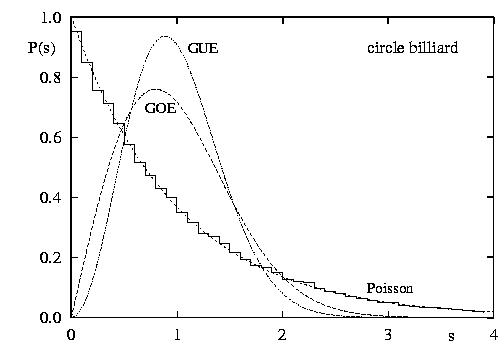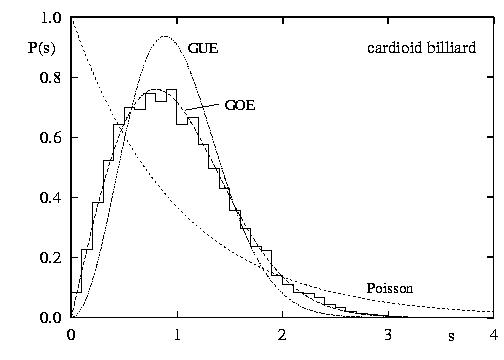The main task in the theory of quantum chaos is to find universal statistical signatures in the quantum energy levels and the distribution of wave intensities of the particle, which correlate with the degree of chaos in the underlying classical dynamics. The figure above shows the distribution of gaps between the energy levels of an electron confined to a circular domain (left) and a heart-shaped domain (right). The classical dynamics in the circular domain is integrable (i.e., non-chaotic), and the gaps between quantum energy levels follow an exponential distribution characteristic for uncorrelated random numbers. On the other hand, the classical dynamics in the cardioid is chaotic, and the statistics are well modelled by the gap distribution of eigenvalues of large matrices chosen at random from the Gaussian Orthogonal Random Matrix Ensemble (GOE).

Below is the image of the intensity distribution of two quantum wave functions (eigenstates) of an electron confined to the cardioid. (Blue corresponds to low, Yellow to medium and Red to high intensity. Courtesy of A. Bäcker, TU Dresden)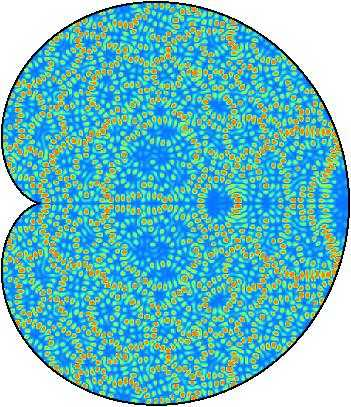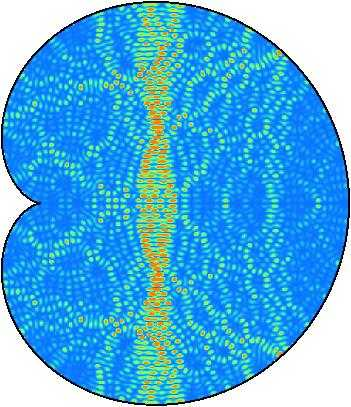One of the central theorems in quantum chaos (Shnirelman's quantum ergodicity theorem) says that, if the underlying classical dynamics is chaotic, then almost all eigenstates look as the one above on the left, in that they are uniformly distributed over the whole domain. Localised eigenstates such as the one above right are very rare and it is not clear whether these still exists at very high energies, i.e., small wave lengths.

The mathematical analysis of systems of the above type is rather involved. Below is a display of two eigenstates of a quantum map which is more accessible to rigorous techniques. The corresponding classical dynamics is here in fact mixed.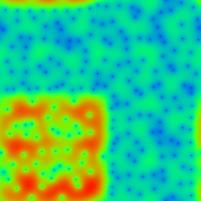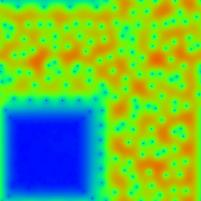The dynamics in the L-shaped region highlighted in the right image is chaotic, while the dynamics in the complement, the small square highlighted on the left, is trivial (i.e., every point is classically mapped to itself). The central result of our research, published in the journal Nonlinearity, is that 25% of all quantum eigenstates localise completely on the small square (the eigenstate on the left is one example), and 75% of all eigenstates are uniformly distributed on the L-shaped region (the eigenstate displayed on the right is one example for this behaviour).

We have also observed some rare eigenstates which localise on the boundary between the chaotic and integrable domain; two examples of such eigenstates are displayed below.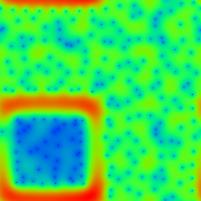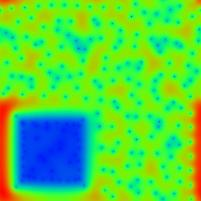The question of the existence of such eigenstates at arbitrarily small wave length remains an interesting challenge for future research. A better understanding of quantum localisation may well have important applications to conductance properties of micro-electronic devices.

For more information see J. Marklof and S. O'Keefe, Weyl's law and quantum ergodicity for maps with divided phase space, with an appendix by S. Zelditch, Nonlinearity 18 (2005) 277-304.